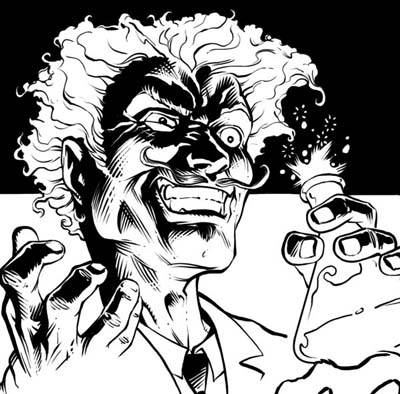# You're mad! Lunatic! Deranged!

Probability Level 3Let $P(n)$ be the probability that a random permutation of $n$ distinct elements have no element in their original position.

Find $\lim_{n \rightarrow \infty} P(n)$

×

Problem Loading...

Note Loading...

Set Loading...